This book is archived and will be removed July 6, 2022. Please use the updated version.

Atomic Structure

# 62 Lasers

### Learning Objectives

By the end of this section, you will be able to:

• Describe the physical processes necessary to produce laser light
• Explain the difference between coherent and incoherent light
• Describe the application of lasers to a CD and Blu-Ray player

A laser is device that emits coherent and monochromatic light. The light is coherent if photons that compose the light are in-phase, and monochromatic if the photons have a single frequency (color). When a gas in the laser absorbs radiation, electrons are elevated to different energy levels. Most electrons return immediately to the ground state, but others linger in what is called a metastable state. It is possible to place a majority of these atoms in a metastable state, a condition called a population inversion.

When a photon of energy disturbs an electron in a metastable state ((Figure)), the electron drops to the lower-energy level and emits an addition photon, and the two photons proceed off together. This process is called stimulated emission. It occurs with relatively high probability when the energy of the incoming photon is equal to the energy difference between the excited and “de-excited” energy levels of the electron (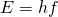). Hence, the incoming photon and the photon produced by de-excitation have the same energy, hf. These photons encounter more electrons in the metastable state, and the process repeats. The result is a cascade or chain reaction of similar de-excitations. Laser light is coherent because all light waves in laser light share the same frequency (color) and the same phase (any two points of along a line perpendicular to the direction of motion are on the “same part” of the wave”). A schematic diagram of coherent and incoherent light wave pattern is given in (Figure).

The physics of a laser. An incident photon of frequency f causes a cascade of photons of the same frequency.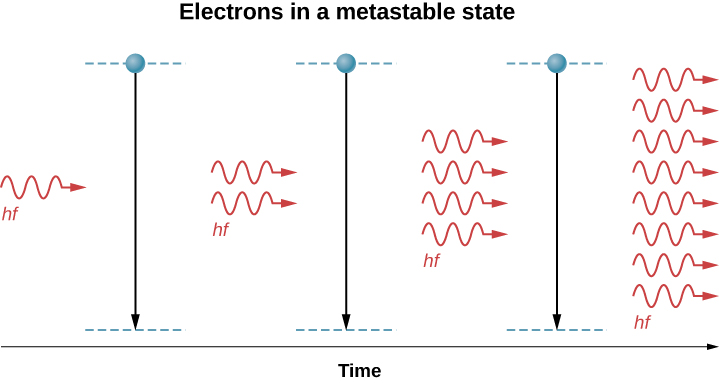A coherent light wave pattern contains light waves of the same frequency and phase. An incoherent light wave pattern contains light waves of different frequencies and phases.Lasers are used in a wide range of applications, such as in communication (optical fiber phone lines), entertainment (laser light shows), medicine (removing tumors and cauterizing vessels in the retina), and in retail sales (bar code readers). Lasers can also be produced by a large range of materials, including solids (for example, the ruby crystal), gases (helium-gas mixture), and liquids (organic dyes). Recently, a laser was even created using gelatin—an edible laser! Below we discuss two practical applications in detail: CD players and Blu-Ray Players.

### CD Player

A CD player reads digital information stored on a compact disc (CD). A CD is 6-inch diameter disc made of plastic that contains small “bumps” and “pits” nears its surface to encode digital or binary data ((Figure)). The bumps and pits appear along a very thin track that spirals outwards from the center of the disc. The width of the track is smaller than 1/20th the width of a human hair, and the heights of the bumps are even smaller yet.

A compact disc is a plastic disc that uses bumps near its surface to encode digital information. The surface of the disc contains multiple layers, including a layer of aluminum and one of polycarbonate plastic.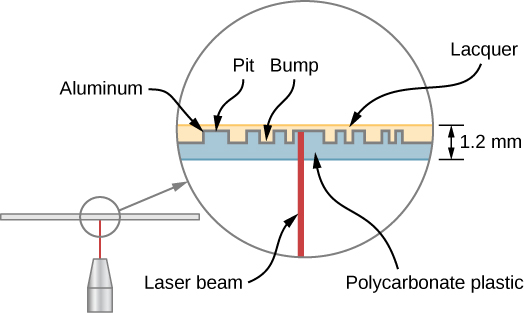A CD player uses a laser to read this digital information. Laser light is suited to this purpose, because coherent light can be focused onto an incredibly small spot and therefore distinguish between bumps and pits in the CD. After processing by player components (including a diffraction grating, polarizer, and collimator), laser light is focused by a lens onto the CD surface. Light that strikes a bump (“land”) is merely reflected, but light that strikes a “pit” destructively interferes, so no light returns (the details of this process are not important to this discussion). Reflected light is interpreted as a “1” and unreflected light is interpreted as a “0.” The resulting digital signal is converted into an analog signal, and the analog signal is fed into an amplifier that powers a device such as a pair of headphones. The laser system of a CD player is shown in (Figure).

A CD player and its laser component.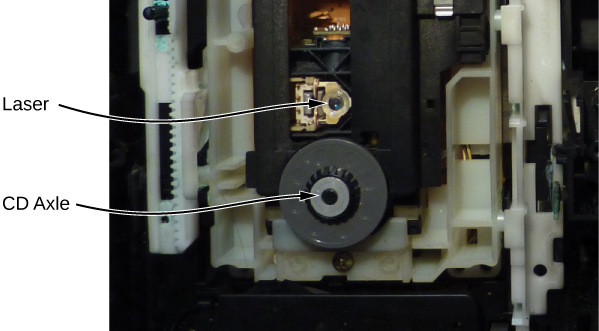### Blu-Ray Player

Like a CD player, a Blu-Ray player reads digital information (video or audio) stored on a disc, and a laser is used to record this information. The pits on a Blu-Ray disc are much smaller and more closely packed together than for a CD, so much more information can be stored. As a result, the resolving power of the laser must be greater. This is achieved using short wavelength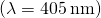blue laser light—hence, the name “Blu-” Ray. (CDs and DVDs use red laser light.) The different pit sizes and player-hardware configurations of a CD, DVD, and Blu-Ray player are shown in (Figure). The pit sizes of a Blu-Ray disk are more than twice as small as the pits on a DVD or CD. Unlike a CD, a Blu-Ray disc store data on a polycarbonate layer, which places the data closer to the lens and avoids readability problems. A hard coating is used to protect the data since it is so close to the surface.

Comparison of laser resolution in a CD, DVD, and Blu-Ray Player.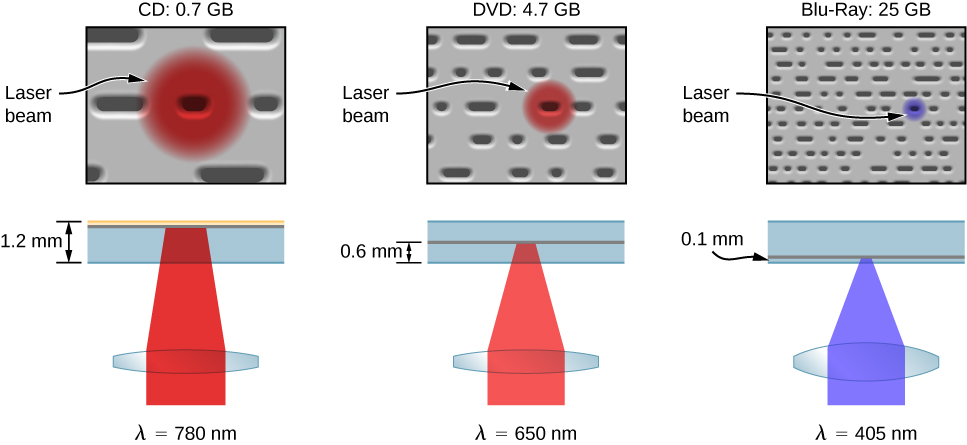### Summary

• Laser light is coherent (monochromatic and “phase linked”) light.
• Laser light is produced by population inversion and subsequent de-excitation of electrons in a material (solid, liquid, or gas).
• CD and Blu-Ray players uses lasers to read digital information stored on discs.

### Key Equations

 Orbital angular momentum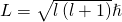z-component of orbital angular momentum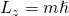Radial probability density function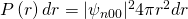Spin angular momentum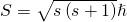z-component of spin angular momentum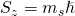Electron spin magnetic moment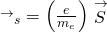Electron orbital magnetic dipole moment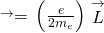Potential energy associated with the magneticinteraction between the orbital magnetic dipolemoment and an external magnetic field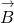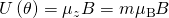Maximum number of electrons in a subshell ofa hydrogen atom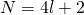Selection rule for atomic transitions in ahydrogen-like atom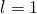Moseley’s law for X-ray production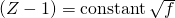### Conceptual Questions

Distinguish between coherent and monochromatic light.

Why is a metastable state necessary for the production of laser light?

Laser light relies on the process of stimulated emission. In this process, electrons must be prepared in an excited (upper) metastable state such that the passage of light through the system produces de-excitations and, therefore, additional light.

How does light from an incandescent light bulb differ from laser light?

A Blu-Ray player uses blue laser light to probe the bumps and pits of the disc and a CD player uses red laser light. The relatively short-wavelength blue light is necessary to probe the smaller pits and bumps on a Blu-ray disc; smaller pits and bumps correspond to higher storage densities.

What are the similarities and differences between a CD player and a Blu-Ray player?

### Problems

A carbon dioxide laser used in surgery emits infrared radiation with a wavelength of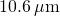. In 1.00 ms, this laser raised the temperature of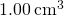of flesh to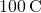and evaporated it. (a) How many photons were required? You may assume that flesh has the same heat of vaporization as water. (b) What was the minimum power output during the flash?

a. If flesh has the same density as water, then we used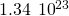photons. b. 2.52 MW

An excimer laser used for vision correction emits UV radiation with a wavelength of 193 nm. (a) Calculate the photon energy in eV. (b) These photons are used to evaporate corneal tissue, which is very similar to water in its properties. Calculate the amount of energy needed per molecule of water to make the phase change from liquid to gas. That is, divide the heat of vaporization in kJ/kg by the number of water molecules in a kilogram. (c) Convert this to eV and compare to the photon energy. Discuss the implications.

For a hydrogen atom in an excited state with principal quantum number n, show that the smallest angle that the orbital angular momentum vector can make with respect to the z-axis is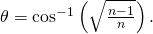The smallest angle corresponds to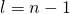and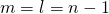. ThereforeWhat is the probability that the 1s electron of a hydrogen atom is found between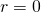and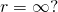Sketch the potential energy function of an electron in a hydrogen atom. (a) What is the value of this function at? in the limit that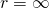? (b) What is unreasonable or inconsistent with the former result?

a. According to (Figure), when,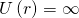, and when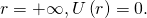b. The former result suggests that the electron can have an infinite negative potential energy. The quantum model of the hydrogen atom avoids this possibility because the probability density atis zero.

Find the value of, the orbital angular momentum quantum number, for the Moon around Earth.

Show that the maximum number of orbital angular momentum electron states in the nth shell of an atom is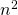. (Ignore electron spin.) (Hint: Make a table of the total number of orbital angular momentum states for each shell and find the pattern.)

A formal solution using sums is somewhat complicated. However, the answer easily found by studying the mathematical pattern between the principal quantum number and the total number of orbital angular momentum states.
For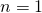, the total number of orbital angular momentum states is 1; for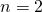, the total number is 4; and, when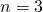, the total number is 9, and so on. The pattern suggests the total number of orbital angular momentum states for the nth shell is.
(Later, when we consider electron spin, the total number of angular momentum states will be found to be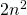, because each orbital angular momentum states is associated with two states of electron spin; spin up and spin down).

What is the magnitude of an electron magnetic moment?

What is the maximum number of electron states in the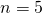shell?

50

A ground-state hydrogen atom is placed in a uniform magnetic field, and a photon is emitted in the transition from a spin-up to spin-down state. The wavelength of the photon is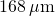. What is the strength of the magnetic field?

Show that the maximum number of electron states in the nth shell of an atom is.

The maximum number of orbital angular momentum electron states in the nth shell of an atom is. Each of these states can be filled by a spin up and spin down electron. So, the maximum number of electron states in the nth shell is.

The valence electron of chlorine is excited to a 3p state. (a) What is the magnitude of the electron’s orbital angular momentum? (b) What are possible values for the z-component of angular measurement?

Which of the following notations are allowed (that is, which violate none of the rules regarding values of quantum numbers)? (a)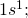(b)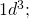(c)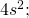(d)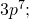(e)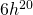a., c., and e. are allowed; the others are not allowed. b.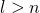is not allowed.
d.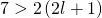The ion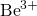makes an atomic transition from anstate to anstate. (a) What is the energy of the photon emitted during the transition? (b) What is the wavelength of the photon?

The maximum characteristic X-ray photon energy comes from the capture of a free electron into a K shell vacancy. What is this photon frequency for tungsten, assuming that the free electron has no initial kinetic energy?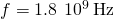Derive an expression for the ratio of X-ray photon frequency for two elements with atomic numbers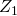and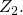Compare the X-ray photon wavelengths for copper and silver.

The atomic numbers for Cu and Ag are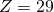and 47, respectively. The X-ray photon frequency for silver is greater than copper by the following factor: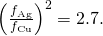Therefore, the X-ray wavelength of Ag is about three times shorter than for copper.

(a) What voltage must be applied to an X-ray tube to obtain 0.0100-fm-wavelength X-rays for use in exploring the details of nuclei? (b) What is unreasonable about this result? (c) Which assumptions are unreasonable or inconsistent?

A student in a physics laboratory observes a hydrogen spectrum with a diffraction grating for the purpose of measuring the wavelengths of the emitted radiation. In the spectrum, she observes a yellow line and finds its wavelength to be 589 nm. (a) Assuming that this is part of the Balmer series, determine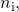the principal quantum number of the initial state. (b) What is unreasonable about this result? (c) Which assumptions are unreasonable or inconsistent?

a. 3.24; b.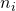is not an integer. c. The wavelength must not be correct. Because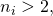the assumption that the line was from the Balmer series is possible, but the wavelength of the light did not produce an integer value for. If the wavelength is correct, then the assumption that the gas is hydrogen is not correct; it might be sodium instead.

### Glossary

coherent light
light that consists of photons of the same frequency and phase
laser
coherent light produced by a cascade of electron de-excitations
metastable state
state in which an electron “lingers” in an excited state
monochromatic
light that consists of photons with the same frequency
population inversion
condition in which a majority of atoms contain electrons in a metastable state
stimulated emission
when a photon of energy triggers an electron in a metastable state to drop in energy emitting an additional photon